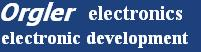View osci data
1 Create this program step by step with Visualc++.net or VisualC++ 6.0The data in the file are only a long list of floats, as you can see in the list on the right We skip the first 4 lines and then we scan line by line info: if your data file uses commas instead of dots, you must convert all commas to dots if you don't have tektronix oscilloscope to save a data file, download this file: download the osci data file (zipped 9Kbyte) ```10000 2e-07 -356.9 0 -0.23 -0.22 -0.22 -0.24 -0.23 -0.23 -0.25 -0.22 -0.21 -0.23 -0.24 -0.21 -0.24 -0.24 .... .... ```
• Create a MFC project named osci and choose SDI "Single Document" Maximized

• Open file osciView.cpp and search:
• `void CosciView::OnDraw(CDC* /*pDC*/)`
• Change this line into:
• `void CosciView::OnDraw(CDC* pDC)`
info: with VisualC++ 6.0 this line needs no change

• Add code to have the same function as below

• Compile and see the axis
• ```void CosciView::OnDraw(CDC* pDC)
{
CosciDoc* pDoc = GetDocument();
ASSERT_VALID(pDoc);
if (!pDoc)
return;
// TODO: add draw code for native data here
#define x0 50
#define y0 400

pDC -> MoveTo(x0,y0);				  // X axis
pDC -> LineTo(x0+1000,y0);

pDC -> MoveTo(x0,y0 - 300);			 // Y axis
pDC -> LineTo(x0,y0 + 300);

// ==>>  code 01
}
```
• open the file osciDoc.cpp

• insert the variable iFilesize and cBuffer

• info: these are global variables
```
// osciDoc.cpp : implementation of the CosciDoc class
#include "stdafx.h"
#include "osci.h"
#include "osciDoc.h"

#ifdef _DEBUG
#define new DEBUG_NEW
#endif

unsigned int iFileSize;
char cBuffer;

```
• now insert the code in function: Serialize

• insert only one line
```void CosciDoc::Serialize(CArchive& ar)
{
if (ar.IsStoring())
{
// TODO: add storing code here
}
else
{
}
}

```
• Have you tested the program ? Everthing okay?

• In the file osciView.cpp we declare the same variables as in osciDoc.cpp, but as extern

• we need some more variables.
info: you can declare all as class variables in the header files, but
we take the simplest way and use global variables
```// osciView.cpp : implementation of the CosciView class
//

#include "stdafx.h"
#include "osci.h"

#include "osciDoc.h"
#include "osciView.h"

#ifdef _DEBUG
#define new DEBUG_NEW
#endif

#define LF 0x0A
extern unsigned int iFileSize;
extern char cBuffer[];
char *ptr1,*ptr2;
float fosciValue;
int xx,yy;
int ii;

```
• Now we extend the code in function OnDraw

• info: if the cBuffer is empty then iFilesize has the value zero and we return;
otherwise, we set the pointer ptr1 at the beginning of cBuffer and ptr2 at the end
```	// ==>>  code 01
if(iFileSize == 0) return;
ptr1     = cBuffer;
ptr2     = ptr1 + iFileSize;
// ==>>  code 02
```
• We skip the first 4 lines

• info: as long as the value of pointer ptr1 is not equal LineFeed, ptr1 is incremented
at last ptr1 must be incremented to skip LF
```	// ==>>  code 02
ii=4;
while(ii--) 			// skip the first 4 lines
{
while(*ptr1 != LF ) ptr1++;	// search Line Feed
ptr1++;				// skip Line Feed
}
// ==>>  code 03

```
• Now we can insert the line scan code and draw the osci data

• info: first we set the pen with MoveTo onto the origin of the axis
we set the X-Axis index xx to zero
we remain in the loop until ptr1(beginning of cBuffer) is lower than ptr2 (end of cBuffer)
The function sscanf is a very old C function and always works very well.
Multiply with a factor and then the value is saved as integer into the variable yy
Now we can draw with LineTo a line from the last point
As there are too many data we always skip 10 lines
```	// ==>>  code 03
pDC -> MoveTo(x0,y0);
xx=0;
while(ptr1 < ptr2)
{
sscanf(ptr1,"%f",&fosciValue);
fosciValue *= 200;
yy = (int)fosciValue;
pDC->LineTo(x0+xx,y0 - yy); xx++;

ii=10;
while(ii--) {	while(*ptr1 != LF ) ptr1++;   ptr1++; } // skip 10 lines
}
```
• If everything is okay, start the program and open the file TEK00000.DAT
• ```void CosciView::OnDraw(CDC* pDC)
{
CosciDoc* pDoc = GetDocument();
ASSERT_VALID(pDoc);
if (!pDoc)
return;
// TODO: add draw code for native data here
#define x0 50
#define y0 400

pDC -> MoveTo(x0,y0);				  // X axis
pDC -> LineTo(x0+1000,y0);

pDC -> MoveTo(x0,y0 - 300);			  // Y axis
pDC -> LineTo(x0,y0 + 300);

// ==>>  code 01
if(iFileSize == 0) return;
ptr1     = cBuffer;
ptr2     = ptr1 + iFileSize;
// ==>>  code 02

ii=4;
while(ii--) {	while(*ptr1 != LF ) ptr1++;   ptr1++; } // skip the first 4 lines

// ==>>  code 03
pDC -> MoveTo(x0,y0);
xx=0;
while(ptr1 < ptr2)
{
sscanf(ptr1,"%f",&fosciValue);
fosciValue *= 200;
yy = (int)fosciValue;
pDC->LineTo(x0+xx,y0 - yy); xx++;

ii=10;
while(ii--) {	while(*ptr1 != LF ) ptr1++;   ptr1++; } // skip 10 lines
}
}
```